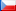### Seeking for help in Electrical measurements

• Posted at : 1 month ago
• Post Similar Project
30

Budget
22
Proposals
186
Views
Expired
Status
Skills Required

Posted By -

TYCzech Republic
0.0
Projects Posted : 1
Projects Paid : 0
Services Purchased : 0
Total Spent :
0
Feedbacks : 0 %

#### Project Details hide (-)

Hello! I need help in answering these question. They have to be fully algebraically derived. Topic: electrical engineering, electrical measurements.

26. Equivalent circuits of resistors, inductors and capacitors.

27. AC Wheatstone bridge. General equation of balanced state. Schering
bridge - a scheme and the derivation of Cx and Rx.

28. Digital measurement of impedance and admittance (using operational
amplifier and vector voltmeter), measurements of impedance of inductors or capacitors.
Scheme and derivation.
29. Transducers for measuring of a sum of voltages. Scheme and
evaluating the relationship between input and output voltages.

30. Integration amplifier. Scheme and evaluating the relationship
between input and output voltages.

31. Hall probe. Scheme and principal.

32. Ferromagnetic probe. Drawing a scheme and derivation of the
relationship between the intensity of mag. field and output voltage.

33. Measuring air coil. Scheme a derivation of the relationship between
the intensity of mag. field and output voltage.

34. Measurement of characteristics of ferromagnetic materials. DC
magnetization of closed sample (toroid). Scheme and derive a relationship for H
and ΔB.

35. Digital to analog converters. Example 4-bit DAC. Scheme and deriving
the output voltage depending on the values ​​of individual bits.

36. Sampling circuit. Scheme and explain using a graph two time
constants τ1 and τ2 and what requirements they impose.

37. Compensation ADC. Draw a scheme and explain the algorithm using the
output voltage in each iteration.

38. Comparative ADC - parallel comparison (FLASH). Draw a diagram and
explain the principle.

39. Integration ADC with dual-integration. Draw a diagram and explain
the working principle.

40. Integration ADC with voltage-frequency conversion. Draw a diagram
and explain the working principle.

41. Digital storage oscilloscopes (digital oscilloscope). Draw a diagram
and explain the working principle.

42. Analog function generator of harmonic, saw tooth and square wave
voltage. Diagram and explain the principle.

43. Generator programmable waveform. Draw a diagram and explain a
working principle.

44. Stabilization of amplifier gain using negative feedback. Scheme and
derivation of the gain.

45. Inverting amplifier. Schema and derivation of the amplifier gain,
relationship for the input and output impedance.

46. ​​The non-inverting amplifier. Schema and derivation of the relationship
for the input and output impedance.

47. The current to voltage converter. Schema, conversion relationship,
relationship for the input and output impedance.

48. The voltage to current converter. Schema, conversion relationship,
relationship for the input and output impedance.

49. Digital frequency meter. Direct method for measurement of frequency.
Draw a diagram and explain the working principle.

50. Determining the frequency (period) of sampled signal. Explain on a waveform
how to increase a precision of the method.